# determinant

(redirected from cryptodeterminant)
Also found in: Dictionary, Thesaurus, Medical.

## determinant,

a polynomial expression that is inherent in the entries of a square matrixmatrix,
in mathematics, a rectangular array of elements (e.g., numbers) considered as a single entity. A matrix is distinguished by the number of rows and columns it contains. The matrix

is a 2×3 (read "2 by 3") matrix, because it contains 2 rows and 3 columns.
..... Click the link for more information.
. The size n of the square matrix, as determined from the number of entries in any row or column, is called the order of the determinant. If the entry in row i and column j is denoted as aij, then, for n=2, the determinant is a11a22a12a21. For example, the second-order determinant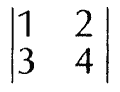has the value (1×4)−(2×3)=4−6=−2. Its absolute valueabsolute value,
magnitude of a number or other mathematical expression disregarding its sign; thus, the absolute value is positive, whether the original expression is positive or negative.
..... Click the link for more information.
is the area of the parallelogram spanned by its row vectors. Third order determinants are similarly related to volumes. A determinant of order n is indicated by |aij|, where i and j each take on the values 1, 2, 3, … n. Its non-vanishing detects invertibility of the matrix. Its value is the sum of all terms S(π)a1π(1)anπ(n), where π ranges over all permutations of (1, 2, … n) and S(π)=±1 is a sign called the signature of π. This value may be found more easily by expanding the determinant by minors. The minor Aij of an element aij of an nth-order determinant is the determinant of order (n−1) formed by deleting the ith row and the jth column of the original determinant. For example, in the determinant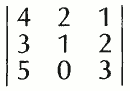the element a21, whose value is 3, has the minor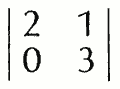In expanding a determinant by minors, first the minor of every element in a particular row or column is formed. Products are derived by multiplying each minor by its corresponding element. A plus sign is placed in front of each product if the sum of the row number and column number of its element is even, and a minus sign if the sum is odd. Finally, the signed products are added algebraically. For example, expanding the above determinant by its second row yields: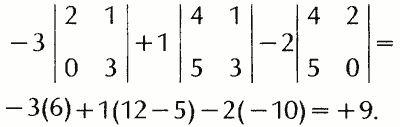Determinants of higher order can be evaluated by successive expansions of this type. By choosing rows of columns containing zeros, some terms can be eliminated. There are various rules for transforming a given determinant, which can be used to obtain a row or column most of whose elements are zeros. Determinants have many applications in mathematics and other fields, e.g., in the solution of simultaneous linear equations.

The Columbia Electronic Encyclopedia™ Copyright © 2013, Columbia University Press. Licensed from Columbia University Press. All rights reserved. www.cc.columbia.edu/cu/cup/
The following article is from The Great Soviet Encyclopedia (1979). It might be outdated or ideologically biased.

## Determinant

a type of function encountered in various branches of mathematics. Consider a matrix of order n, that is, a square array of n2 elements, for example, numbers or functions: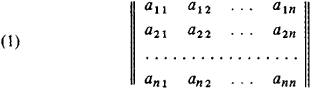Each element of the matrix is specified by two indices. The element aij belongs to the i th row and the j th column. The determinant of the matrix (1) is a polynomial in the entries aij;

∑ ±aaa

In this formula, α, β, …, γ is an arbitrary permutation of the numbers 1,2, …, n. The plus or minus sign is used according to whether the permutation α, β, …, γ is even or odd. (A permutation is even if it contains an even number of inversions, that is, cases in which a larger number precedes a smaller number; otherwise, the permutation is odd. Thus, for example, the permutation 51243 is odd, since it contains five inversions, namely, 51, 52, 54, 53, 43.) The summation extends over all permutations α, β, ‖, γ of the numbers 1, 2, …, n. Thus only one element from any row and any column enters each product. The number of distinct permutations of n symbols is n! = 1 · 2 · 3 · … ·n; therefore, a determinant contains n! terms, of which ½n! have a plus sign and ½n! have a minus sign. The number n is called the order of the determinant. The determinant of the matrix (1) is written as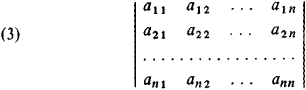or, briefly, ǀaikǀ. For determinants of order 2 and 3, we have the formulas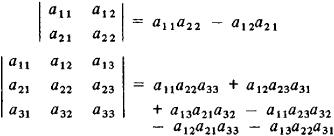Determinants of order 2 and 3 admit a simple geometric interpretation:is (except possibly for sign) the area of the parallelogram on the vectors a1 = (x1, y1) and a2 = (x2, y2),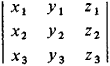and is (except possibly for sign) the volume of the parallelepiped on the vectors a1 = (x1, y1, z1), a2 = (x2, y2, z2), and a3 = (x3, y3, z3). In each case it is assumed that the coordinate system is rectangular.

The theory of determinants arose in connection with the problem of solving a system of first-degree algebraic equations (linear equations). In the most important case, when the number of equations equals the number of unknowns, such a system may be written in the formThis system has a unique solution if the determinant ǀaikǀ of the coefficients of the unknowns is different from zero; in that case, the unknown xm (m = 1, 2, …, n) is a fraction whose denominator is the determinant ǀaikǀ and whose numerator is the determinant obtained from ǀaikǀ by replacing the elements of the mth column, that is, the coefficients of xm, by the numbers b1, b2, …, bn. Thus, in the case of a system of two equations in two unknownsthe solution is given by the formulas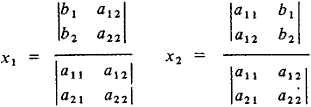If b1 = b1 = … = bn = 0, then (4) is called homogeneous. A homogenous system has nonzero solutions only if ǀaikǀ = 0. The connection between the theory of determinants and the theory of linear equations enables us to apply the theory of determinants to the solution of a large number of problems in analytic geometry. Many formulas of analytic geometry can conveniently be written using determinants; for example, the equation of the plane passing through the points with coordinates (x1, y1, z1), (x2, y2, Z2), and (x3, y3, z3) can be written in the formDeterminants have a number of important properties, some of which facilitate their computation. Below we list the simplest of these properties.

(1) A determinant does not change if its rows and columns are interchanged: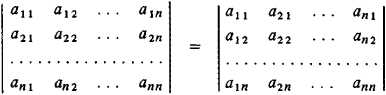(2) A determinant changes sign if two of its rows or two of its columns are interchanged; thus, for example,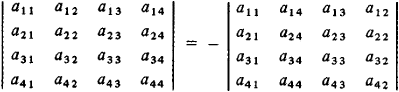(3) A determinant is equal to zero if the elements in two of its rows or columns are proportional; thus, for example,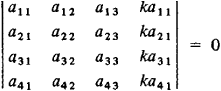(4) A factor common to all the elements of a row or column of a determinant can be placed outside the determinant; thus, for example,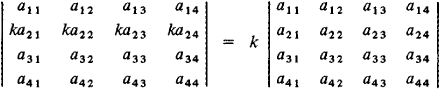(5) If each element in some column (row) of a determinant is a sum of two terms, then the determinant is the sum of two determinants, in one of which the corresponding column (row) consists of the first terms and in the other the corresponding column (row) consists of the second terms, while the remaining columns (rows) are the same as in the original determinant; thus for example,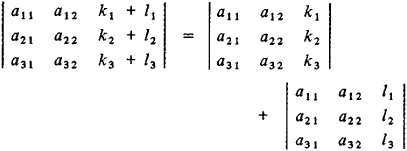(6) A determinant does not change if the elements of one row (column) are multiplied by an arbitrary constant and added to the elements of another row (column); thus, for example,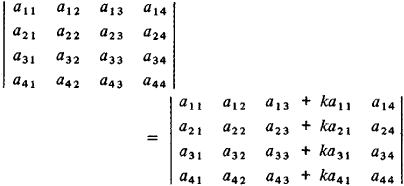(7) A determinant can be expanded by the elements of any row or any column. The expansion of the determinant (3) by the elements of the i th row has the form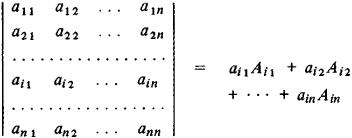The coefficient Aik of aik is called the cofactor of aik The cofactor Aik = (– 1)i + kDik, where Dik is the minor associated with the element an, that is, the determinant of order n – 1 obtained from the original determinant by crossing out the i th row and the k th column. For example, the expansion of a determinant of order 3 by the elements of the second column is given by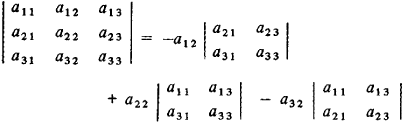Expansion of a determinant of order n by a row or column reduces computation of the determinant to the computation of n determinants of order n — 1. Thus the computation of a determinant of order 5, say, reduces to the computation of five determinants of order 4; the computation of each of these determinants of order 4 can, in turn, be reduced to the computation of four determinants of order 3 (the formula for the computation of a determinant of order 3 has been given above). However, except for the simplest cases, this method of computing determinants is practical only for determinants of relatively low order. For the computation of determinants of high order, a number of more convenient methods have been developed (approximately n3 operations must be performed to compute a determinant of order n).

An important rule is the rule for multiplying two determinants of order n. The product of two determinants of order n may be expressed as a single determinant of order n in which the element belonging to the i th row and the k th column is obtained by first multiplying each element in the i th row of the first factor by the corresponding element in the k th column of the second factor and then summing all these products. In other words, the product of the determinants of two matrices is the determinant of the product of these matrices.

Determinants have been systematically used in mathematical analysis ever since the second quarter of the 19th century, when the German mathematician K. Jacobi studied determinants in which the elements are not numbers but functions of one or more variables. The most interesting of these determinants is the Jacobian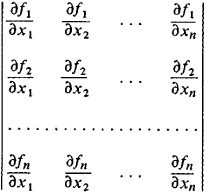The Jacobian gives the local value of the factor by which volumes are altered by the change of variables

y1 = f1(x1, …, xn)

y2 = f2(x1, …, xn)

.................................

yN = fn(x1, …, xn)

The vanishing of this determinant in a certain region is a necessary and sufficient condition for the functional dependence of the functions f1(x1,…,xn), f2(x1, …,xn), …, fn (x1, …,xn) in that region.

The theory of determinants of infinite order was developed in the second half of the 19th century. Infinite determinants are expressions of the type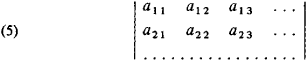(one-sided infinite determinant) or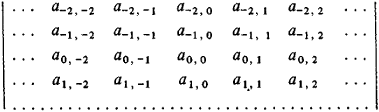(two-sided infinite determinant). The infinite determinant (5) is the limit of the determinantas n → ∞. If this limit exists, then the determinant is called convergent; otherwise, it is divergent. The study of a two-sided infinite determinant may sometimes be reduced to the study of a certain one-sided infinite determinant.

The theory of determinants of finite order was created mainly in the second half of the 18th century and the first half of the 19th century by the Swiss mathematician G. Cramer, the French mathematicians A. Vandermonde, P. Laplace, and A. Cauchy, and the German mathematicians K. Gauss and Jacobi. The term “determinant” was proposed by Gauss, and the modern notation is due to the British mathematician A. Cayley.

### REFERENCES

See references under LINEAR ALGEBRA and .
The Great Soviet Encyclopedia, 3rd Edition (1970-1979). © 2010 The Gale Group, Inc. All rights reserved.

## determinant

[də′tər·mə·nənt]
(control systems)
The product of the partial return differences associated with the nodes of a signal-flow graph.
(mathematics)
A certain real-valued function of the column vectors of a square matrix which is zero if and only if the matrix is singular; used to solve systems of linear equations and to study linear transformations.
McGraw-Hill Dictionary of Scientific & Technical Terms, 6E, Copyright © 2003 by The McGraw-Hill Companies, Inc.

## determinant

Maths a square array of elements that represents the sum of certain products of these elements, used to solve simultaneous equations, in vector studies, etc.
Collins Discovery Encyclopedia, 1st edition © HarperCollins Publishers 2005
Site: Follow: Share:
Open / Close